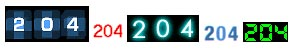# Page hit counter by using digit images for graphical display

We will try to create graphical or attractive digit counter by using our PHP counter script. The purpose of this script is to display images like below in place of simple displaying the text of numbers.We will not discuss how the counter should work, how the data is to be read and written to the text file. You can read the counter script file to get the details. We will start from the incremented data or the data, which we are displaying as, counter value and work on it to display image counter.

In that script the variable \$contents store the value of the present value of the counter. We will start from there.

Here to display a graphical counter we have to call the digits. We will have graphical digits from 0 to 9 in different styles stored in different directories. To display in particular style we will call the digits of the directory.

To display the number 502 we will call or display the digit 5.gif then 0.gif and then 2.gif. This will make the graphical counter. Same way to display 758 counter value we will call first 7.gif then we will call 5.gif and then we will display 8.gif. We have kept different style of digits in different directories so by changing the directory name we can change the style of the digits.

Now let us find out how to know what digit to display by reading the number. There can be many ways here. One is to use string functions to identify the digits in the number. We will use mathematical functions to do that here. You can develop your own way to do this also. As our number system is of base 10 so dividing a number by 10 and collecting the reminder will give us the right most digit. So if we divide 502 by 10 then we will get 2 as reminder and we can store it in an array. Then the quotient can again be divided by 10 to get the next number( 50/ 10 will give reminder 0 ) and we will do this till we get all the digits. Here is the code to do this.

### \$cdisp=\$contents; // Storing the counter value in another variable\$divisor=10; // setting the divisor value to 10\$digitarray=array(); // creating an array do {\$digit=(\$cdisp % \$divisor); // looping through the till all digits are taken\$cdisp=(\$cdisp/\$divisor); // getting the digits from right sidearray_push(\$digitarray,\$digit); // storing them in the array} while(\$cdisp >=1); // condition of do loop// array is to be reversed as digits are in reverse order\$digitarray=array_reverse(\$digitarray); \$dir="d5"; // setting the direcotry value. for different styles while (list (\$key, \$val) = each (\$digitarray)) { // looping through the arrayecho "<img src='digits/\$dir/\$val.gif'>"; // calling one by one digits based on the value of the array} // end of the loop

PHP Page Hit counter

Visitors Rating
Your Rating

GD functions in PHP for handling images & graphicsHow to check GD support in PHPHeader for an image in browserDrawing of graphic lines using GDPlotting line graph by drawing data from tableAdding vertical grid to line graphResizing and adding border to ImagesScript for Watermarking image on the fly using PHP GDScript to generate Captcha using GD with demoGetting Image coordinates ( vertical and horizontal ) of points inside a imagePage Hit counter script

Post your comments , suggestion , error , requirements etc here .

 HTML MySQL PHP JavaScript ASP Photoshop Articles FORUM . Contact us
©2000-2019 plus2net.com All rights reserved worldwide Privacy Policy Disclaimer